# 15 251 Some Great Theoretical Ideas in Computer

• Slides: 53
Download presentation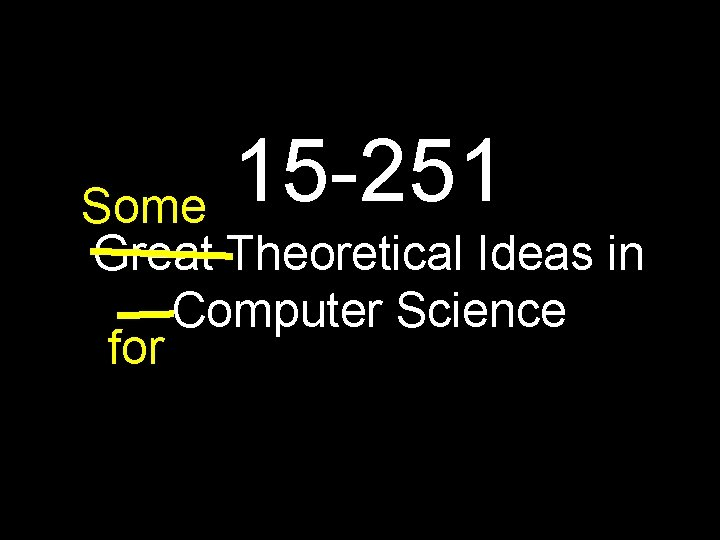15 -251 Some Great Theoretical Ideas in Computer Science forAnupam Gupta Danny Sleator Graphs Lecture 18 (October 21, 2010)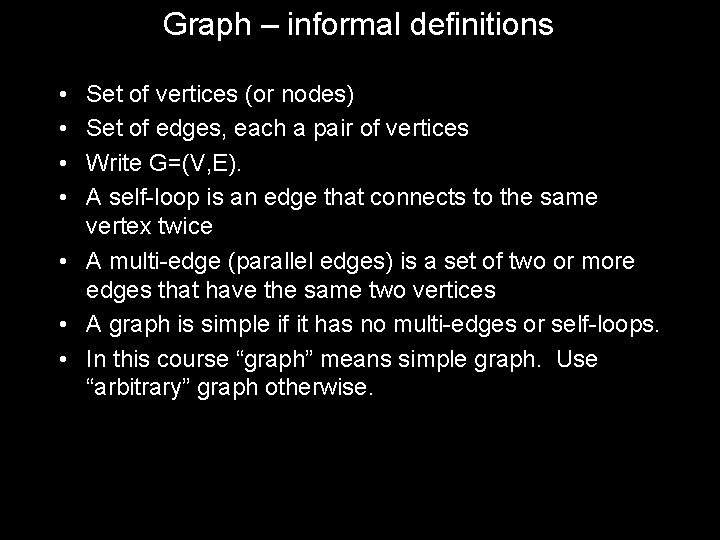Graph – informal definitions • • Set of vertices (or nodes) Set of edges, each a pair of vertices Write G=(V, E). A self-loop is an edge that connects to the same vertex twice • A multi-edge (parallel edges) is a set of two or more edges that have the same two vertices • A graph is simple if it has no multi-edges or self-loops. • In this course “graph” means simple graph. Use “arbitrary” graph otherwise.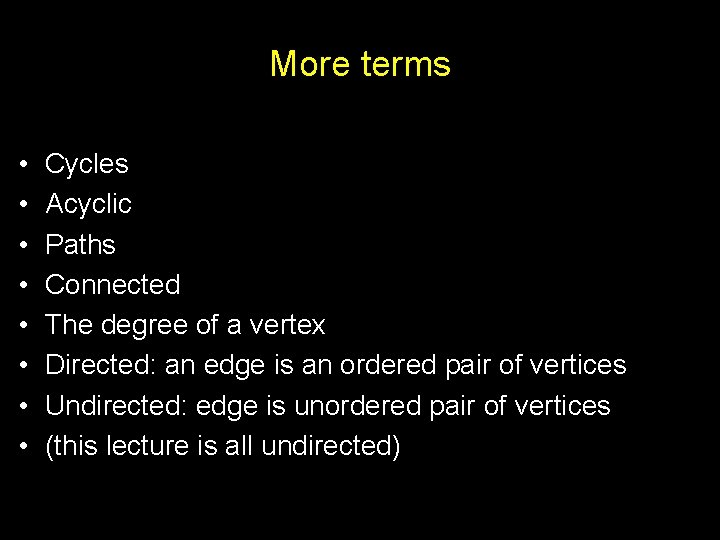More terms • • Cycles Acyclic Paths Connected The degree of a vertex Directed: an edge is an ordered pair of vertices Undirected: edge is unordered pair of vertices (this lecture is all undirected)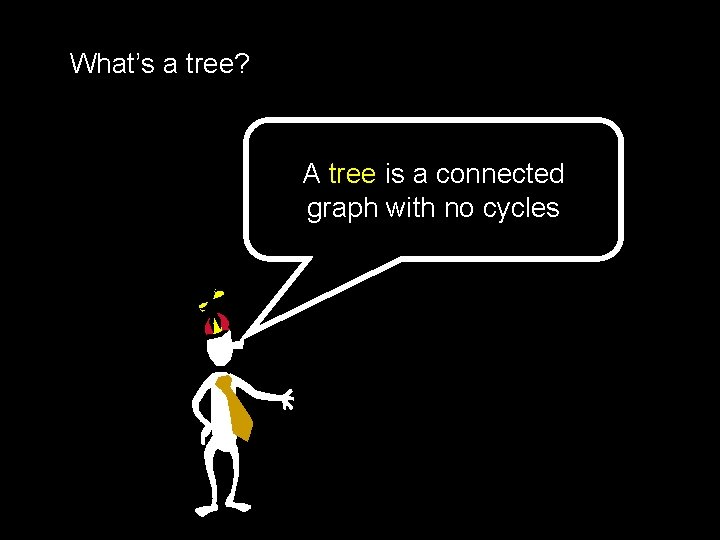What’s a tree? A tree is a connected graph with no cyclesTreeNot TreeNot TreeTreeHow Many n-Node Trees? 1: 2: 3: 4: 5: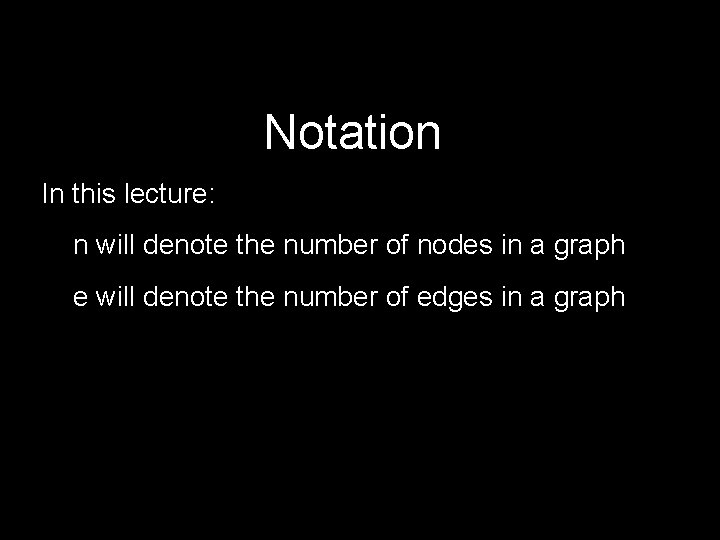Notation In this lecture: n will denote the number of nodes in a graph e will denote the number of edges in a graphTheorem: Let G be a graph with n nodes and e edges The following are equivalent: 1. G is a tree (connected, acyclic) 2. Every two nodes of G are joined by a unique path 3. G is connected and n = e + 1 4. G is acyclic and n = e + 1 5. G is acyclic and if any two non-adjacent nodes are joined by an edge, the resulting graph has exactly one cycle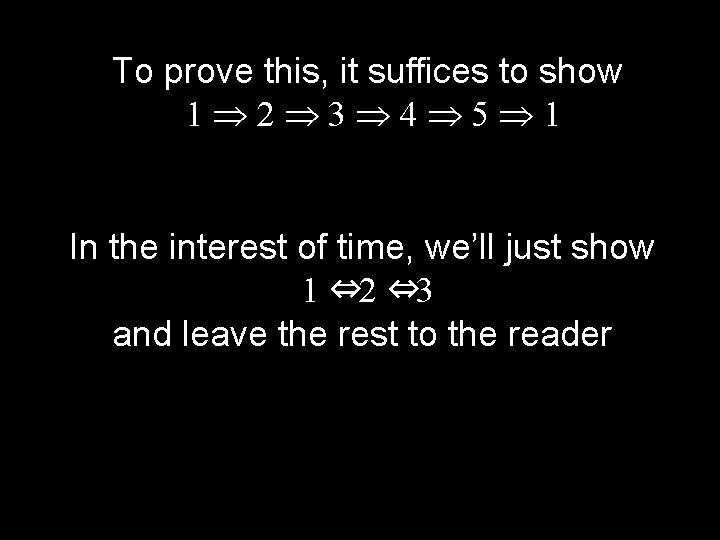To prove this, it suffices to show 1 2 3 4 5 1 In the interest of time, we’ll just show 1 ⇔ 2 ⇔ 3 and leave the rest to the reader1 2 1. G is a tree (connected, acyclic) 2. Every two nodes of G are joined by a unique path Proof: (by contradiction) Assume G is a tree that has two nodes connected by two different paths: Then there exists a cycle!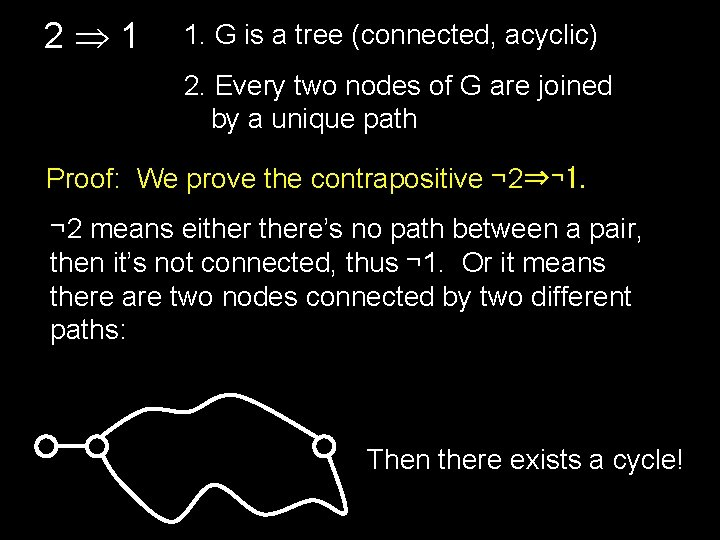2 1 1. G is a tree (connected, acyclic) 2. Every two nodes of G are joined by a unique path Proof: We prove the contrapositive ¬ 2⇒¬ 1. ¬ 2 means eithere’s no path between a pair, then it’s not connected, thus ¬ 1. Or it means there are two nodes connected by two different paths: Then there exists a cycle!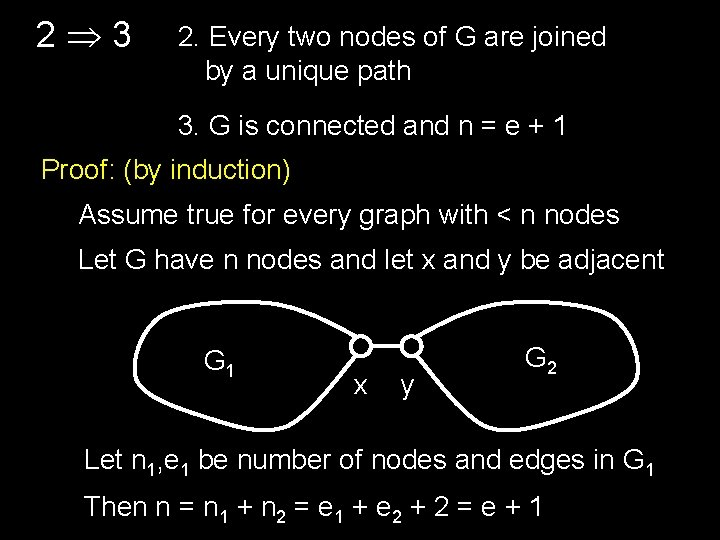2 3 2. Every two nodes of G are joined by a unique path 3. G is connected and n = e + 1 Proof: (by induction) Assume true for every graph with < n nodes Let G have n nodes and let x and y be adjacent G 1 x y G 2 Let n 1, e 1 be number of nodes and edges in G 1 Then n = n 1 + n 2 = e 1 + e 2 + 2 = e + 13 2 2. Every two nodes of G are joined by a unique path 3. G is connected and e = n - 1 Proof: Imagine trying to connect the n nodes of G with n-1 edges, by inserting the edges one by one. The only way to completely connect the graph is if on every step we connect two components together, otherwise we run out of edges. Each component has the unique paths property. x y It’s easy to see that the unique path property holds in the result.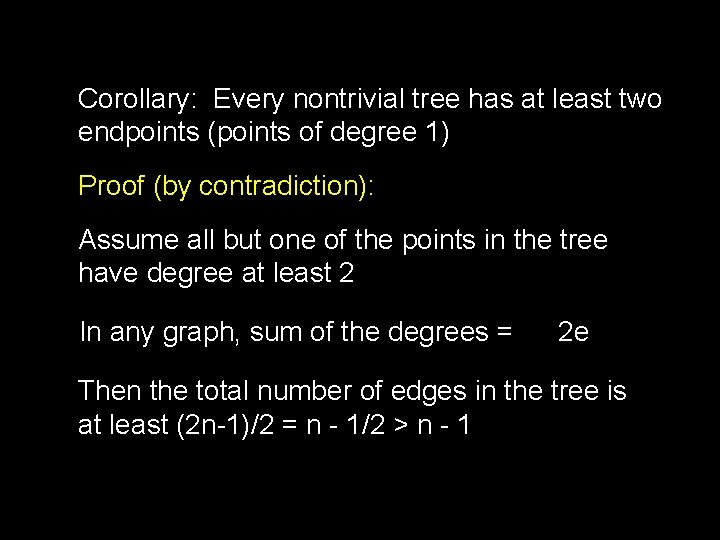Corollary: Every nontrivial tree has at least two endpoints (points of degree 1) Proof (by contradiction): Assume all but one of the points in the tree have degree at least 2 In any graph, sum of the degrees = 2 e Then the total number of edges in the tree is at least (2 n-1)/2 = n - 1/2 > n - 1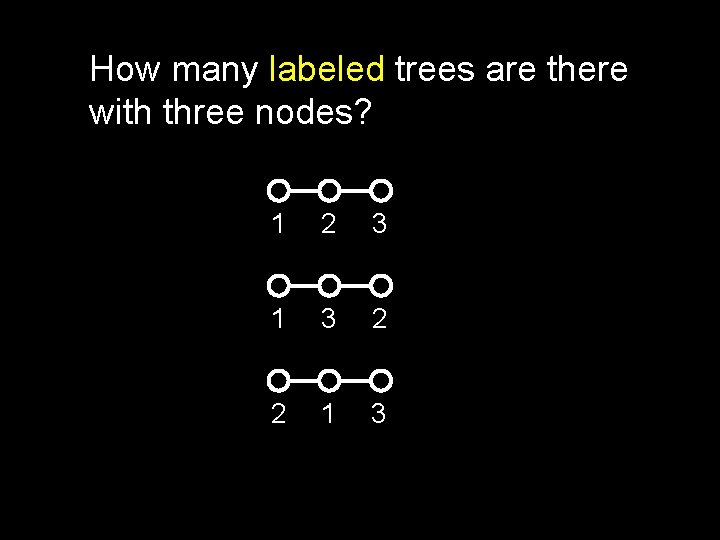How many labeled trees are there with three nodes? 1 2 3 1 3 2 2 1 3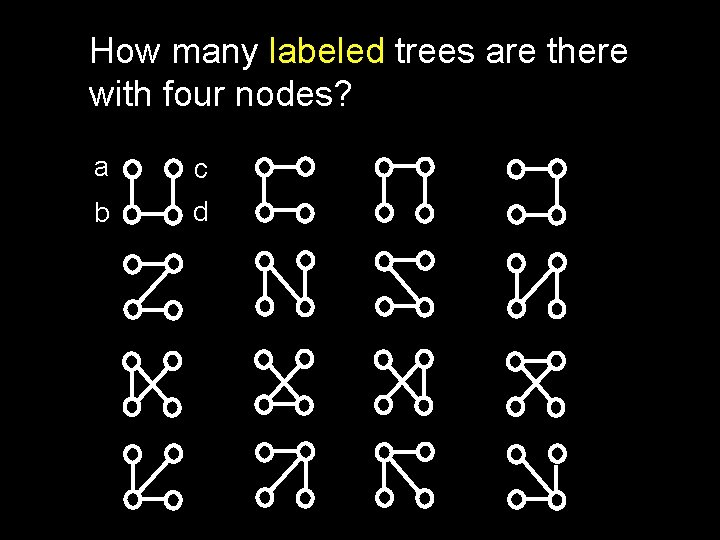How many labeled trees are there with four nodes? a c b d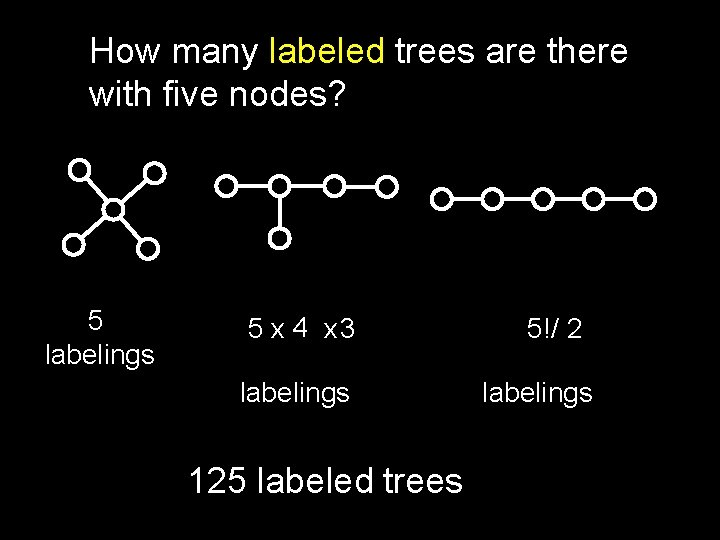How many labeled trees are there with five nodes? 5 labelings 5 x 4 x 3 labelings 125 labeled trees 5!/ 2 labelingsHow many labeled trees are there with n nodes? 3 labeled trees with 3 nodes 16 labeled trees with 4 nodes 125 labeled trees with 5 nodes nn-2 labeled trees with n nodesCayley’s Formula The number of labeled trees on n nodes is nn-2The proof will use the correspondence principle. Let T be the set of labeled n-node trees. Let S be {1, 2, …, n}n-2 (that is, all sequences of n-2 numbers, each in the range [1. . n]) We’ll exhibit a bijection between S and T.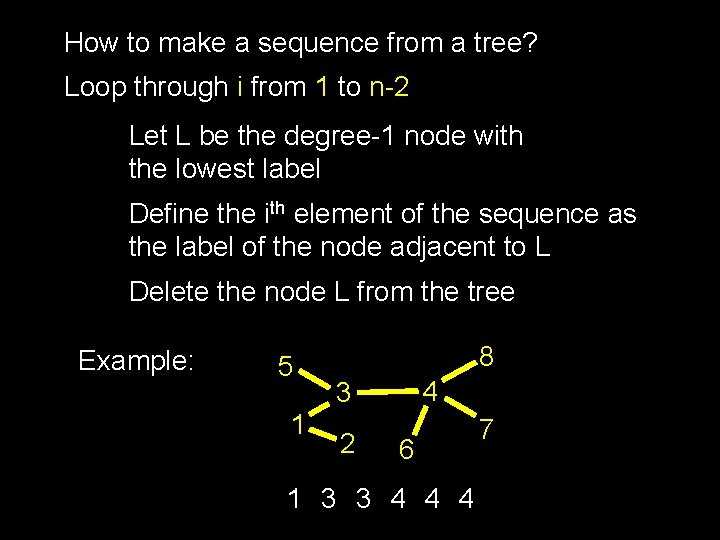How to make a sequence from a tree? Loop through i from 1 to n-2 Let L be the degree-1 node with the lowest label Define the ith element of the sequence as the label of the node adjacent to L Delete the node L from the tree Example: 5 1 8 4 3 2 6 1 3 3 4 4 4 75 1 8 4 3 2 6 7 1 3 3 4 4 4 What can you say about the set of numbers that occur in this sequence? Which nodes are they in the tree?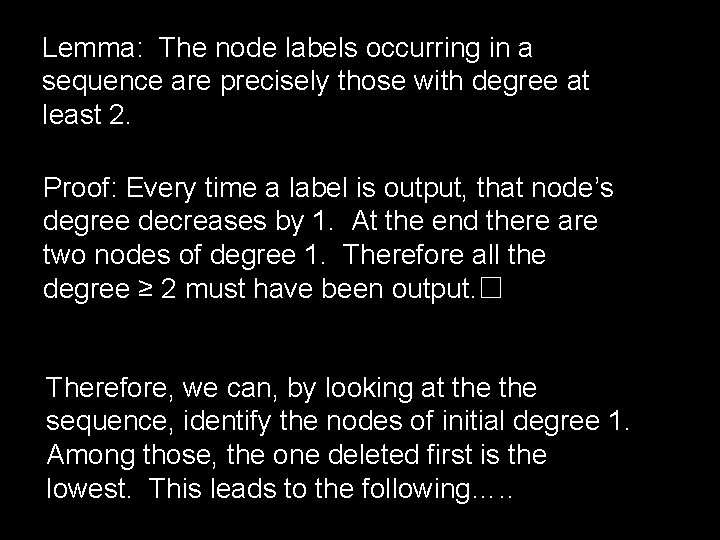Lemma: The node labels occurring in a sequence are precisely those with degree at least 2. Proof: Every time a label is output, that node’s degree decreases by 1. At the end there are two nodes of degree 1. Therefore all the degree ≥ 2 must have been output. ☐ Therefore, we can, by looking at the sequence, identify the nodes of initial degree 1. Among those, the one deleted first is the lowest. This leads to the following…. .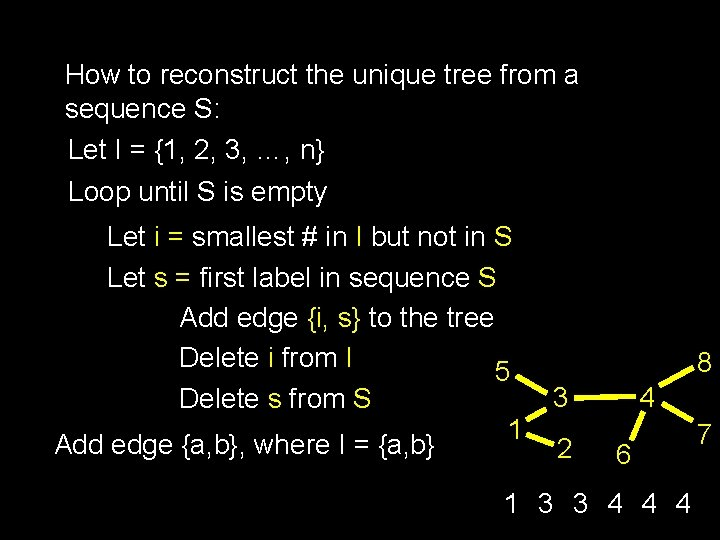How to reconstruct the unique tree from a sequence S: Let I = {1, 2, 3, …, n} Loop until S is empty Let i = smallest # in I but not in S Let s = first label in sequence S Add edge {i, s} to the tree Delete i from I 5 Delete s from S 1 Add edge {a, b}, where I = {a, b} 8 4 3 2 6 1 3 3 4 4 4 7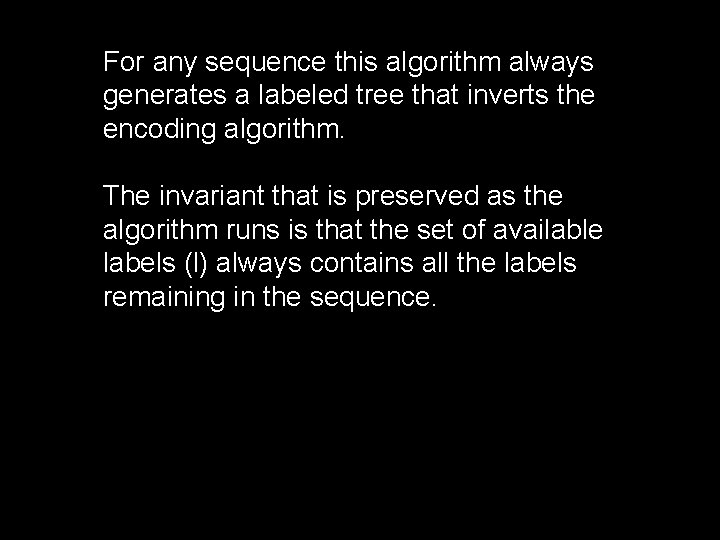For any sequence this algorithm always generates a labeled tree that inverts the encoding algorithm. The invariant that is preserved as the algorithm runs is that the set of available labels (l) always contains all the labels remaining in the sequence.Spanning Trees A spanning tree of a graph G is a tree that touches every node of G and uses only edges from G Every connected graph has a spanning tree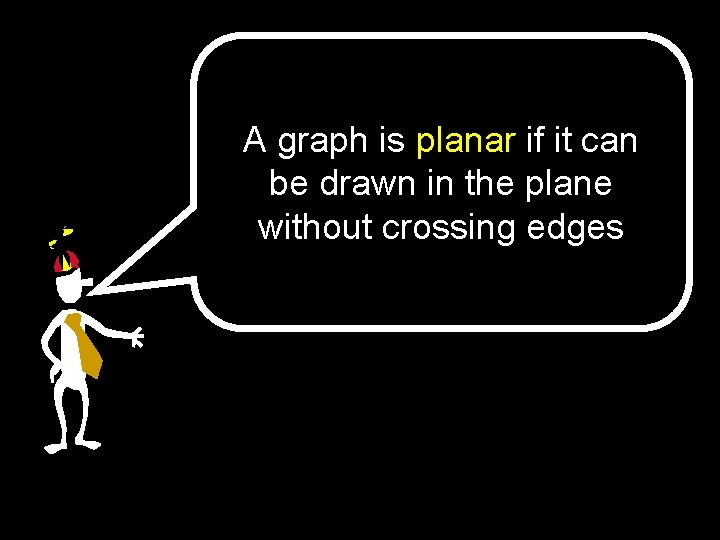A graph is planar if it can be drawn in the plane without crossing edgesExamples of Planar Graphs =http: //www. planarity. net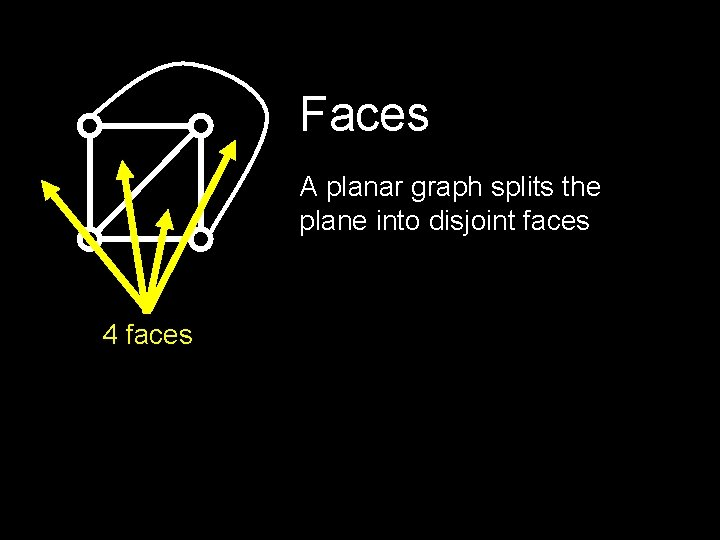Faces A planar graph splits the plane into disjoint faces 4 faces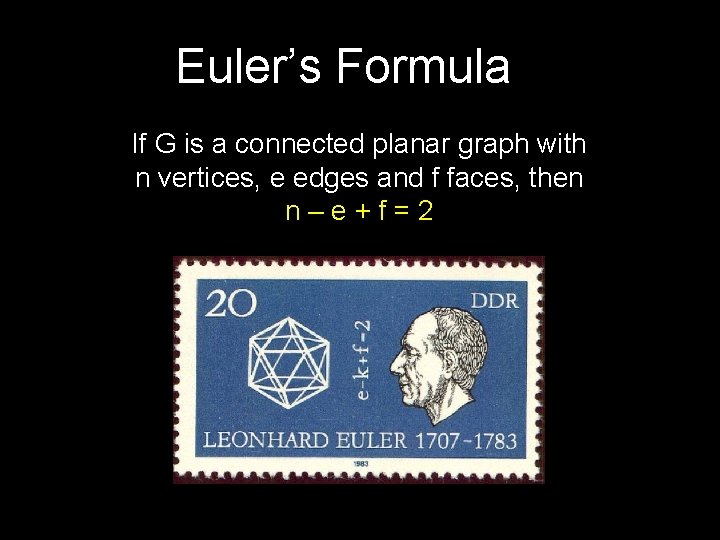Euler’s Formula If G is a connected planar graph with n vertices, e edges and f faces, then n–e+f=2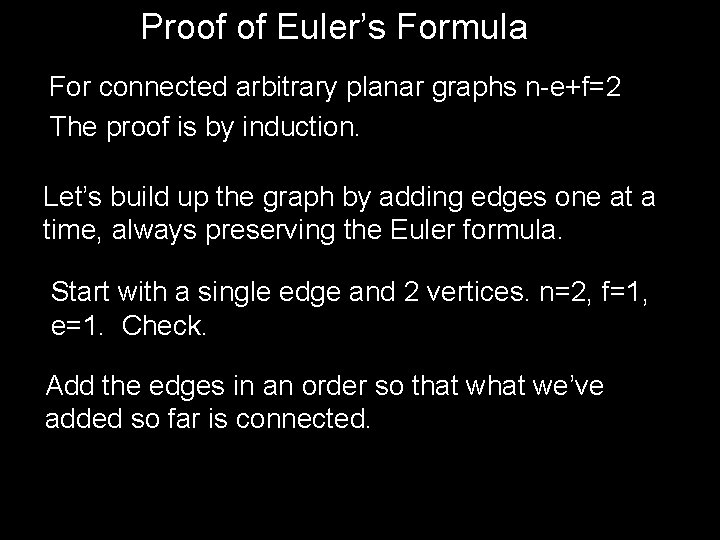Proof of Euler’s Formula For connected arbitrary planar graphs n-e+f=2 The proof is by induction. Let’s build up the graph by adding edges one at a time, always preserving the Euler formula. Start with a single edge and 2 vertices. n=2, f=1, e=1. Check. Add the edges in an order so that we’ve added so far is connected.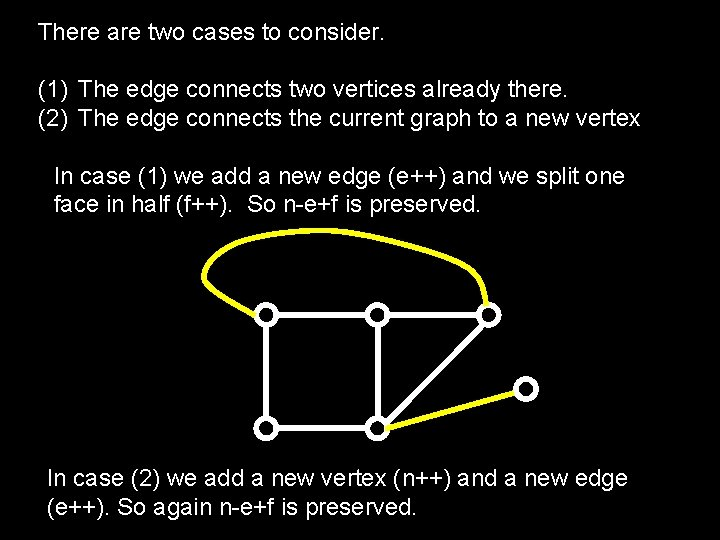There are two cases to consider. (1) The edge connects two vertices already there. (2) The edge connects the current graph to a new vertex In case (1) we add a new edge (e++) and we split one face in half (f++). So n-e+f is preserved. In case (2) we add a new vertex (n++) and a new edge (e++). So again n-e+f is preserved.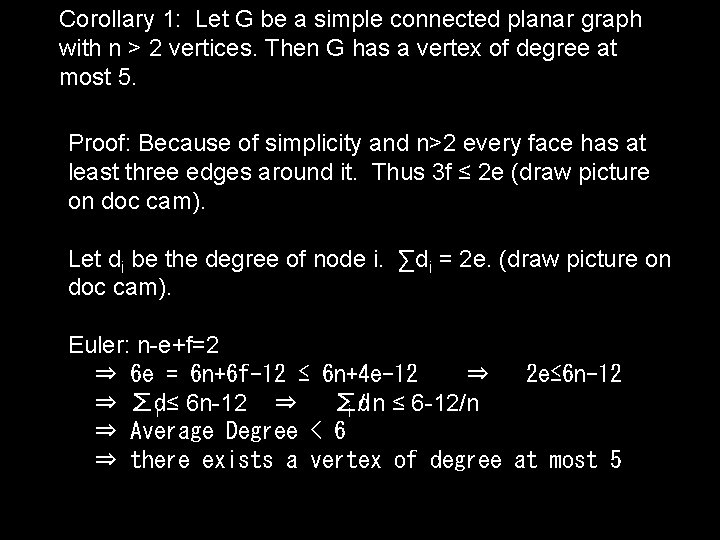Corollary 1: Let G be a simple connected planar graph with n > 2 vertices. Then G has a vertex of degree at most 5. Proof: Because of simplicity and n>2 every face has at least three edges around it. Thus 3 f ≤ 2 e (draw picture on doc cam). Let di be the degree of node i. ∑di = 2 e. (draw picture on doc cam). Euler: n-e+f=2 ⇒ 6 e = 6 n+6 f-12 ≤ 6 n+4 e-12 ⇒ 2 e≤ 6 n-12 ⇒ ∑di ≤ 6 n-12 ⇒ ∑d i / n ≤ 6 -12/n ⇒ Average Degree < 6 ⇒ there exists a vertex of degree at most 5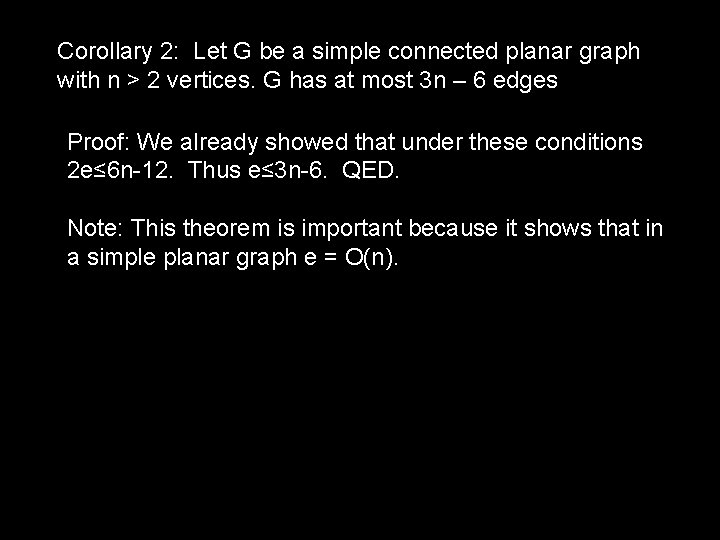Corollary 2: Let G be a simple connected planar graph with n > 2 vertices. G has at most 3 n – 6 edges Proof: We already showed that under these conditions 2 e≤ 6 n-12. Thus e≤ 3 n-6. QED. Note: This theorem is important because it shows that in a simple planar graph e = O(n).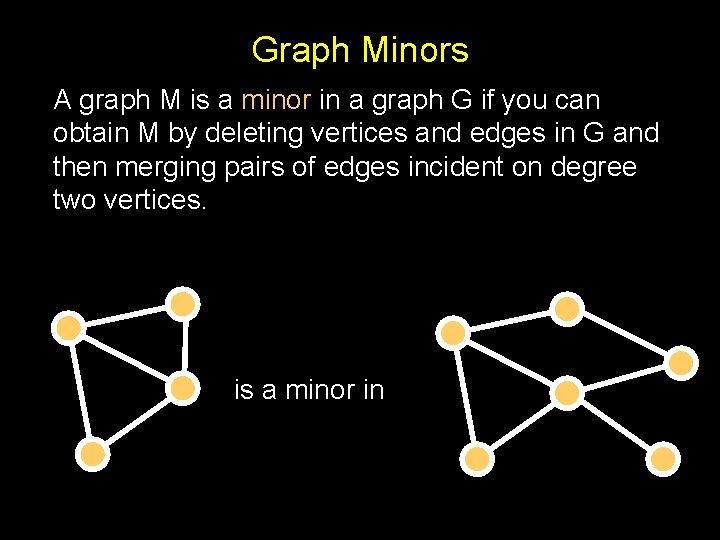Graph Minors A graph M is a minor in a graph G if you can obtain M by deleting vertices and edges in G and then merging pairs of edges incident on degree two vertices. is a minor in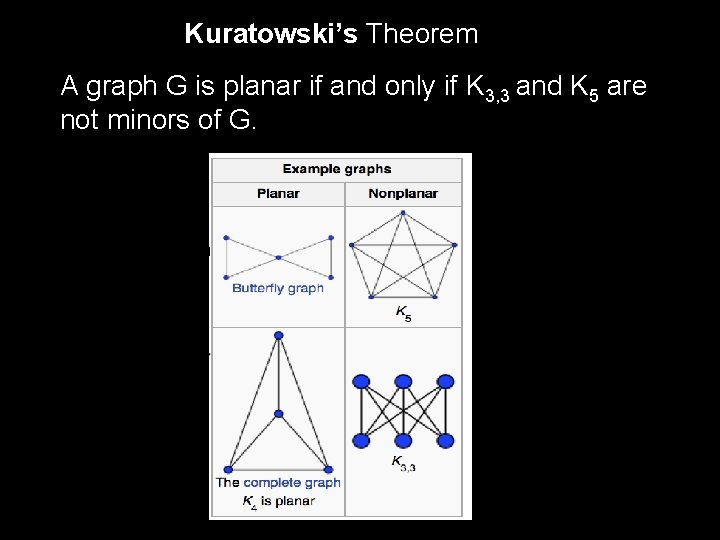Kuratowski’s Theorem A graph G is planar if and only if K 3, 3 and K 5 are not minors of G.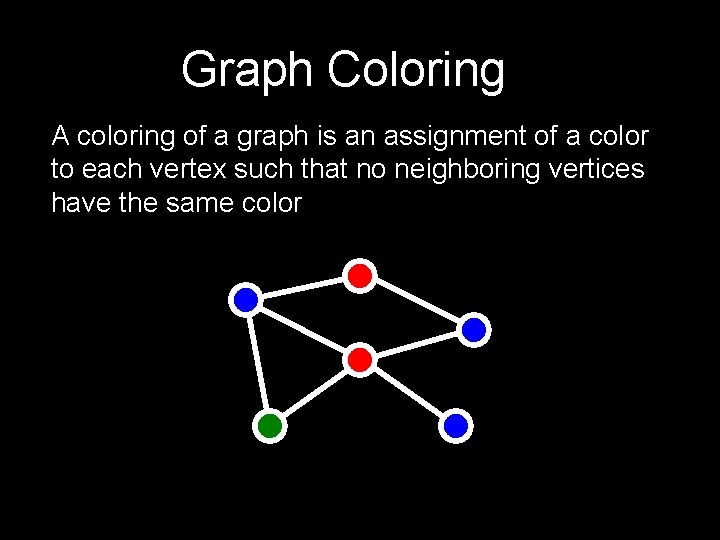Graph Coloring A coloring of a graph is an assignment of a color to each vertex such that no neighboring vertices have the same colorGraph Coloring Arises surprisingly often in CS Register allocation: assign temporary variables to registers for scheduling instructions. Variables that interfere, or are simultaneously active, cannot be assigned to the same registerTheorem: Every planar graph can be 5 -colored Proof (by induction): Assume every planar graph with less than n vertices can be 5 -colored. A base case of n<6 is trivial. Assume G has n vertices Since G is planar, it has some node v with degree at most 5. If deg(v)<5, Remove v and color by with 5 colors. Now color v with a color not used among its neighbors. (A slight technicality here is that we have to keep the graph connected in order to apply the Euler formula. If removal of v disconnects the graph, then you can add sufficient edges among the neighbors of v to retain connectivity. )What if v has degree 5? We know that all pairs of the neighbors of v cannot be edges (they’d form K 5) So there is a pair (v, w) which are not neighbors. We now identify these two vertices as one, and apply the 5 -coloring method to the resulting smaller connected planar graph. Now notice that we can apply this coloring to G, and among the neighbor of v, only 4 colors are used. Thus we can color v with the 5 th color.A computer-assisted proof of the 4 -color theorem was discovered in 1976 by Appel and Haken of the University of Illinois.Implementing Graphs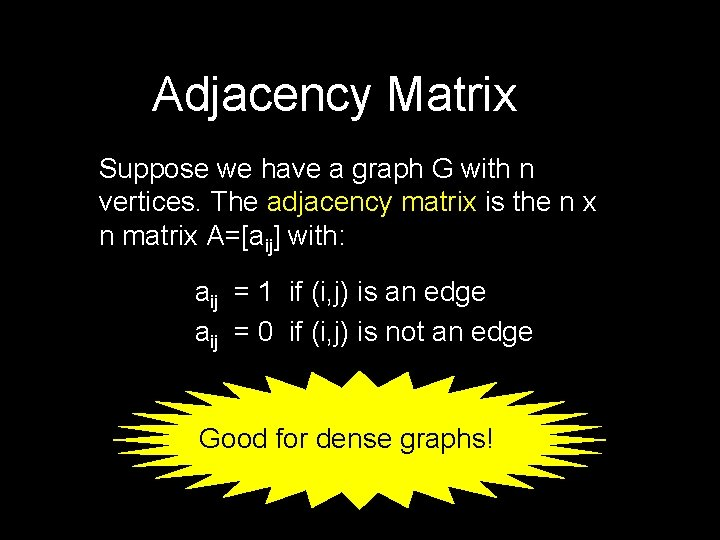Adjacency Matrix Suppose we have a graph G with n vertices. The adjacency matrix is the n x n matrix A=[aij] with: aij = 1 if (i, j) is an edge aij = 0 if (i, j) is not an edge Good for dense graphs!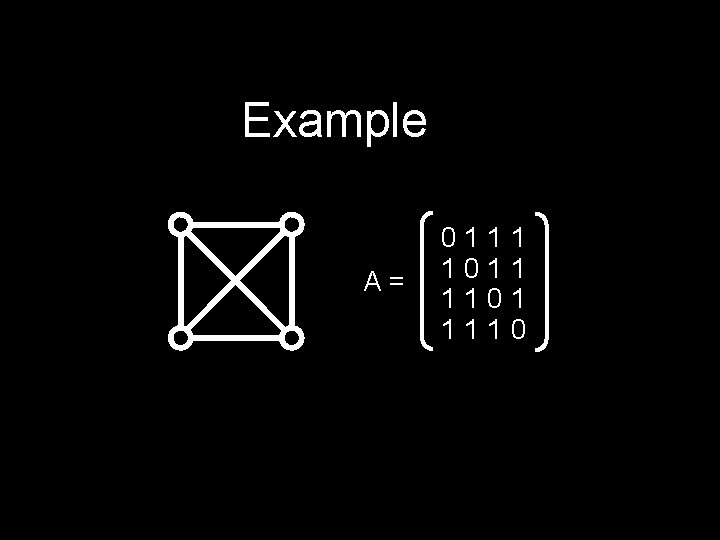Example A= 0111 1011 1101 1110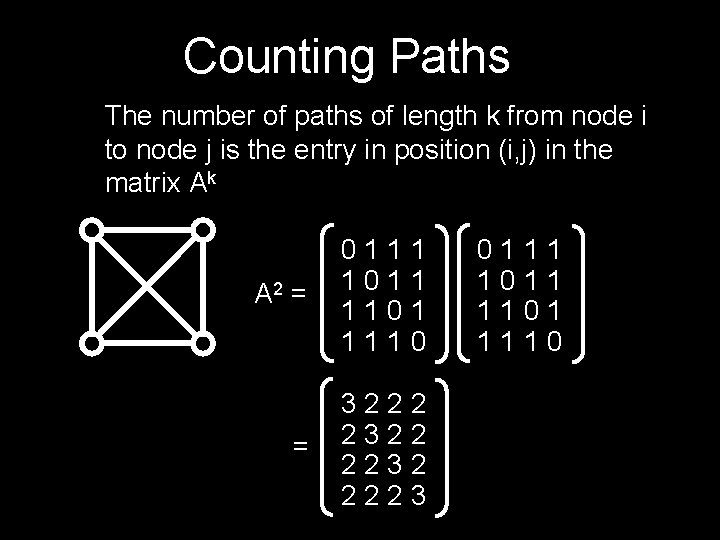Counting Paths The number of paths of length k from node i to node j is the entry in position (i, j) in the matrix Ak A 2 = 0111 1011 1101 1110 = 3222 2322 2232 2223 0111 1011 1101 1110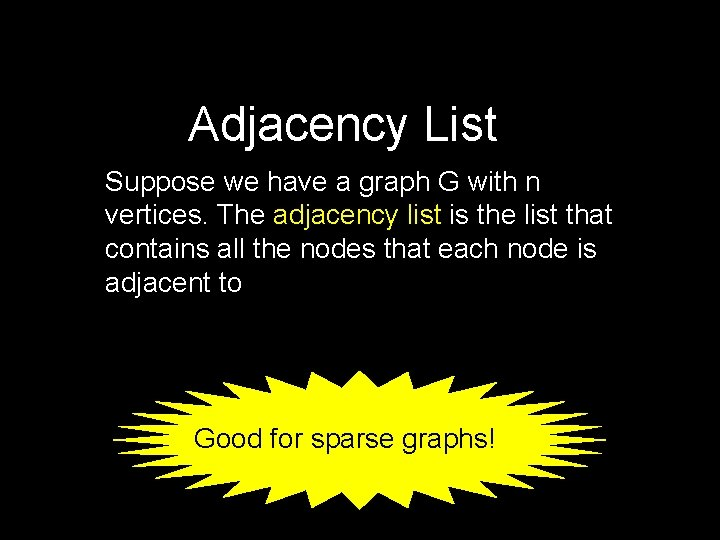Adjacency List Suppose we have a graph G with n vertices. The adjacency list is the list that contains all the nodes that each node is adjacent to Good for sparse graphs!Example 1 3 2 4 1: 2, 3 2: 1, 3, 4 3: 1, 2, 4 4: 2, 3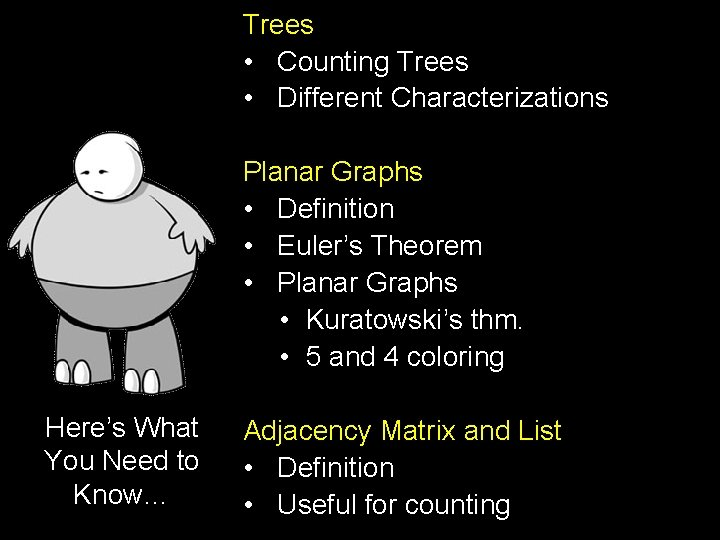Trees • Counting Trees • Different Characterizations Planar Graphs • Definition • Euler’s Theorem • Planar Graphs • Kuratowski’s thm. • 5 and 4 coloring Here’s What You Need to Know… Adjacency Matrix and List • Definition • Useful for counting